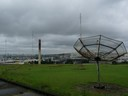Version 1.7 a new dawn

## lecture: From Maxwell to antenna arrays

### How 150 year old equations still help to communicateMaxwell's equations are four differential equations which form the foundation of classical electrodynamics, classical optics, and electric circuits. This talk will take a look at the connection between these equations, wave propagation and antenna arrays.

Maxwell's equations describe how electric and magnetic fields are generated and altered by each other and by charges and currents. They are named after the Scottish physicist and mathematician James Clerk Maxwell, who published those equations exactly 150 years ago and form the foundation of classical electrodynamics. Actually Maxwell had 20 equations and it took another 20 years until they were understood and the self-taught British engineer, mathematician, and physicist Heaviside put the equations in their present form. Some years later Hertz brought the experimental prove of Maxwell's theory.

Under a lot of simplifying boundary conditions wave propagation and antenna theory can be derived from this four equations. This talk will lead from Maxwell's equations to wave equations and nice antenna forms and arrays. Also some practical aspects will be evaluated. Why do low frequencies reach farther than higher frequencies? Why do radio astronomers spread their antenna arrays over whole continents? Or why is China Mobile building antenna arrays for mobile radio consisting of 128 antennas?

### Info

Day: 2014-12-27
Start time: 23:00
Duration: 01:00
Room: Saal G
Track: Science
Language: en